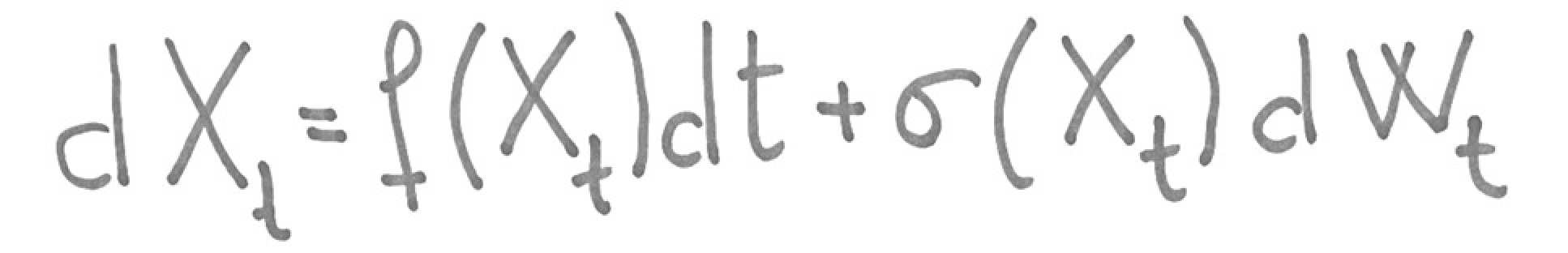ResearchThe research interests of the Stochastic Analysis group cover a wide selection of topics. The common denominator of these topics is the rich interplay between classical analysis and probability theory. The group is interested in many theoretical issues as well as applications to Mathematical Finance, Physics and Engineering problems.  The following is a minute sample of research topics of the group: linear and nonlinear SPDEs, forward-backward SDEs, rough path theory, asymptotic behaviour of stochastic processes, filtering, sequential Monte Carlo methods, particle approximations, statistical methods for stochastic processes.

Applications to Mathematical finance include:  asymptotic methods for robust pricing and calibration of financial derivatives, models of insider trading using initial enlargment of filtrations, valuation and hedging of derivative securities, Stochastic volatility modelling. Credit risk valuation, modelling the dynamics of energy prices.

View our working papers series archive